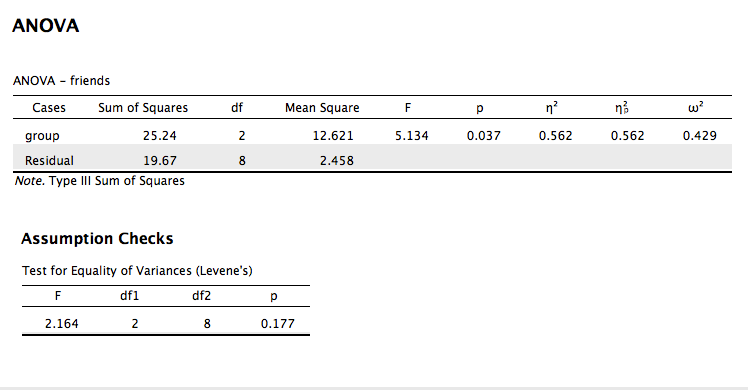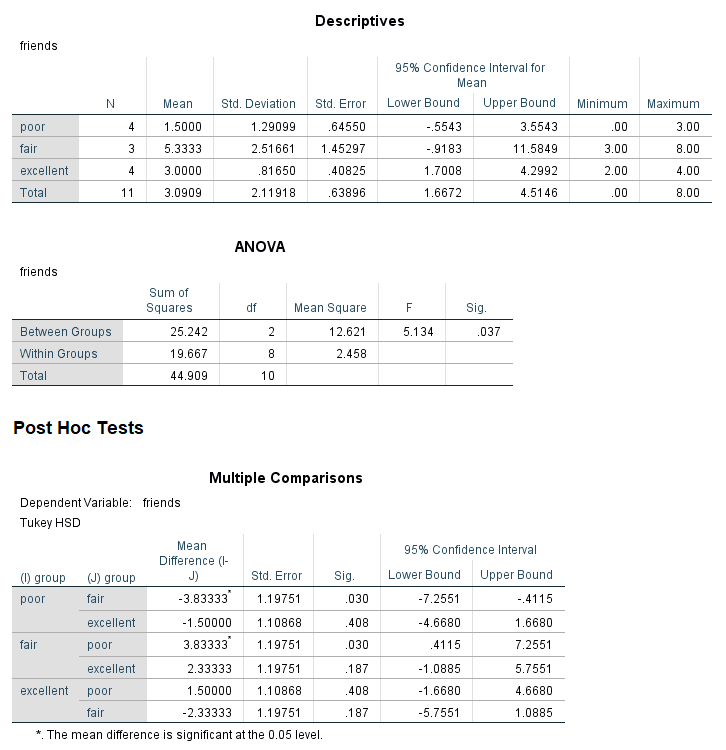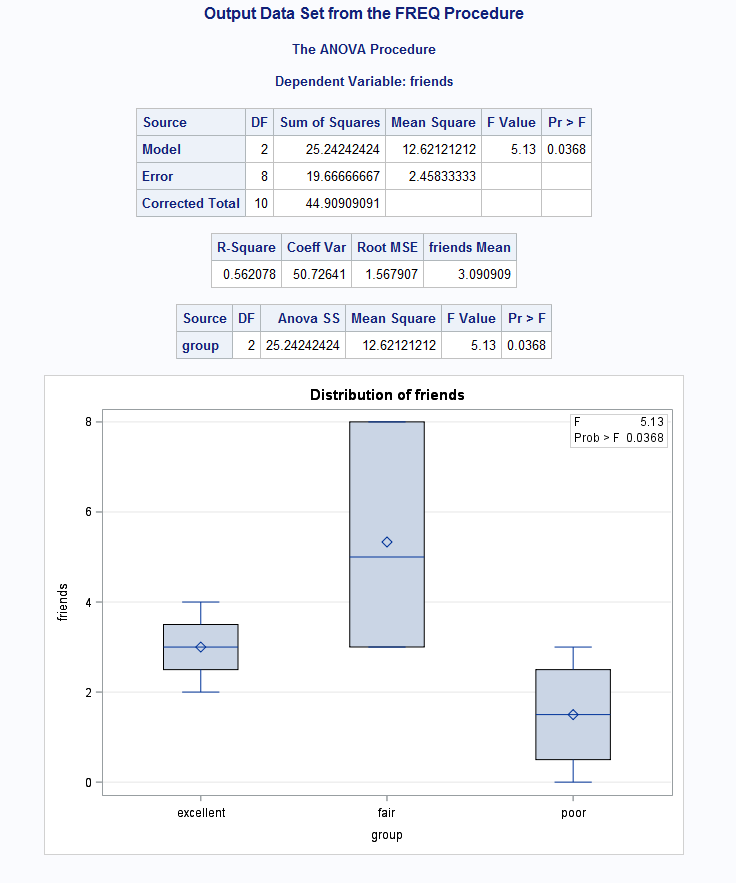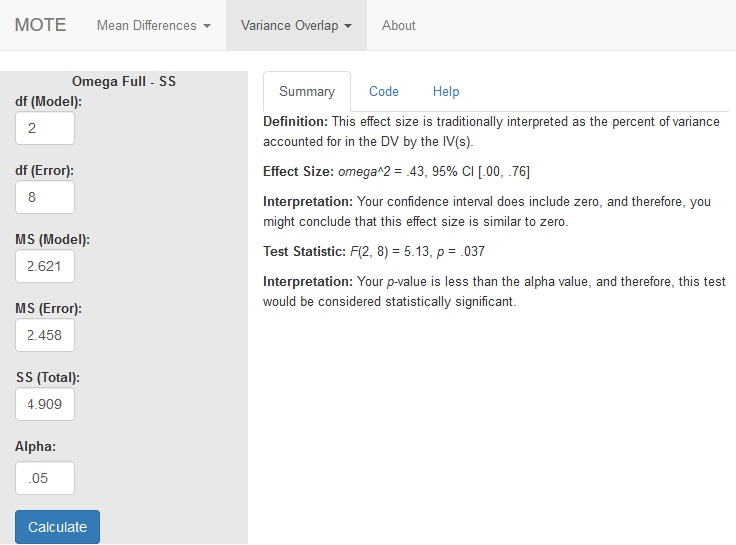# Description

This function displays omega squared from ANOVA analyses and its non-central confidence interval based on the F distribution. This formula works for one way and multi way designs with careful focus on which error term you are using for the calculation.

The formula for $\omega^2$ is: $$\frac{df_{model} \times (MS_{model} - MS_{error})}{SS_{model} + SS_{error} + SS_{subject} + MS_{subject} }$$

The formula for F is: $$\frac{MS_{model}}{MS_{error}}$$

# R Function

omega.full.SS(dfm, dfe, msm, mse, sst, a = 0.05)

# Arguments

• dfm = degrees of freedom for the model/IV/between
• dfe = degrees of freedom for the error/residual/within
• msm = mean square for the model/IV/between
• mse = mean square for the error/residual/within
• sst = sum of squares total
• a = significance level

# Example

A health psychologist recorded the number of close inter-personal attachments of 45-year-olds who were in excellent, fair, or poor health. People in the Excellent Health group had 4, 3, 2, and 3 close attachments; people in the Fair Health group had 3, 5, and 8 close attachments; and people in the Poor Health group had 3, 1, 0, and 2 close attachments. The data are available on GitHub. Example output from JASP, SPSS, and SAS are shown below.

JASPSPSSSAS# Function in R:

omega.full.SS(dfm = 2, dfe = 8, msm = 12.621, mse = 2.458, sst = 44.909, a = 0.05)

# MOTE

## Screenshot## Effect Size:

$\omega^2$ = .43, 95% CI [.00, .76]

## Interpretation:

Your confidence interval does include zero, and therefore, you might conclude that this effect size is similar to zero.

Not applicable.

## Test Statistic:

F(2, 8) = 5.13, p = .037

## Interpretation:

Your p-value is less than the alpha value, and therefore, this test would be considered statistically significant.